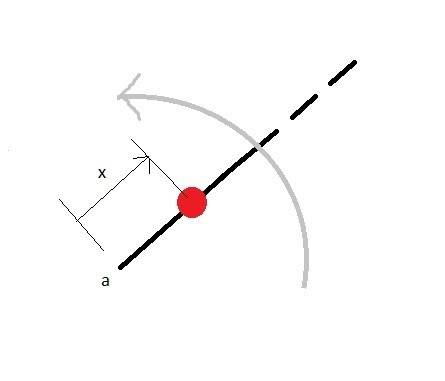# Bead sliding on a rotating straight rigid wire.

## Homework Statement

I think I will start with the figure below:The wire of infinite length rotates about the point "a" with constant angular speed. The bead starts out at rest. There is no friction or gravity.

I have already derived the equations of motion for this system (I used Lagrangian dynamics) and from those equations I know that the bead accelerates radially along the wire (x increases). But what I can't explain is how this bead is moving in a direction in which no force is acting on it.

The only force acting on the bead is the reaction force of the wire on the bead, but that is perpendicular to the wire so it should not cause the bead to move outward along the wire.

The equations tell me the behavior of the bead, but I cannot intuitively understand what is making it move outward along the wire.

## Homework Equations

Let i, j, k be unit vectors. i is along the radius of the wire. j is in tangential direction and k is out of the page/screen.

position vector to bead: r = x*i where i is a unit vector along the radius stuck in the wire.

v = x'*i + cross_product{ w*k, x*i}

v = x'*i + w*x*j

Kinetic Energy T = 0.5 * m *(x'^2 + (w*x)^2 )

Apply Lagrange's equation to obtain:

m*x'' - m*w^2*x = 0

So the bead will accelerate radially.

:(

Last edited:

## Answers and Replies

tiny-tim
Science Advisor
Homework Helper
Hi Aerostd!(try using the X2 icon just above the Reply box)
The only force acting on the bead is the reaction force of the wire on the bead, but that is perpendicular to the wire so it should not cause the bead to move outward along the wire.

The equations tell me the behavior of the bead, but I cannot intuitively understand what is making it move outward along the wire.

Apply Lagrange's equation to obtain:

m*x'' - m*w^2*x = 0

So the bead will accelerate radially.

It only appears to accelerate radially.

For example, if you twirl something in a circle on the end of a rope, it appears to have zero radial acceleration, but as you know it actually has the centripetal acceleration of -ω2r.

This bead has total acceleration -ω2r + r'', which of course is zero.r= A*exp(ωt);
A based on initial position